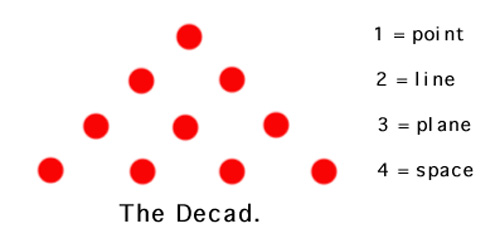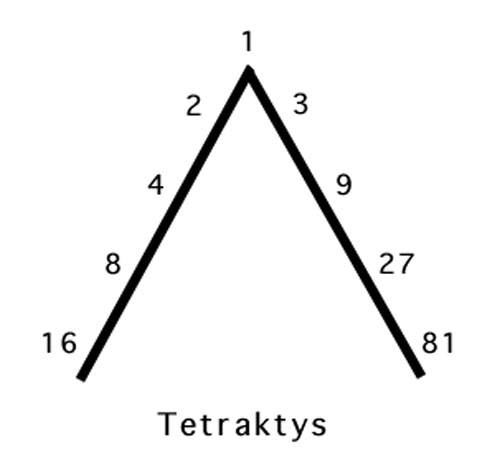# Decad - TetraktysTen was the divine number for the Pythagoreans. They would arrange 10 stones in the order shown above to form the Decad.  Together it comprised the sum of dimensional experience.  Just as 1 + 2 + 3 + 4 = 10.The Tetraktys was another form of dimensional experience for the Pythagoreans.  Its series on each leg of the triangle is decidedly geometric.  Filling in between spaces yield the harmonic ratios.  This is the starting point for a discussion on the Introduction to Arithmetic by Nicomachus of Gerasa, A.D. 100 and quite possibly the best place to start with number theory.